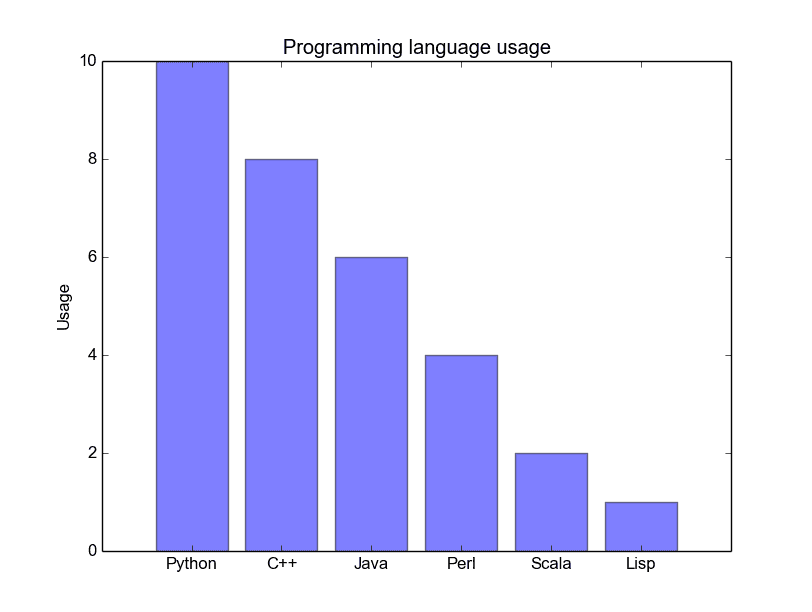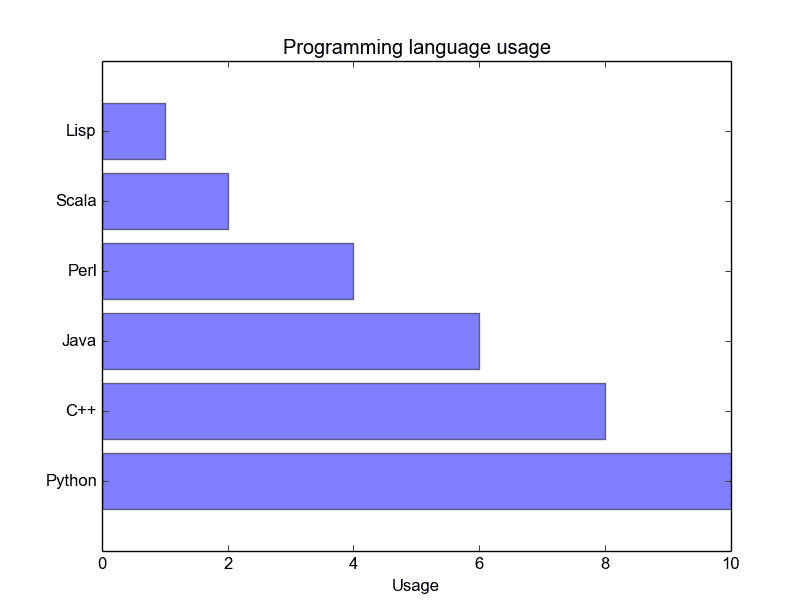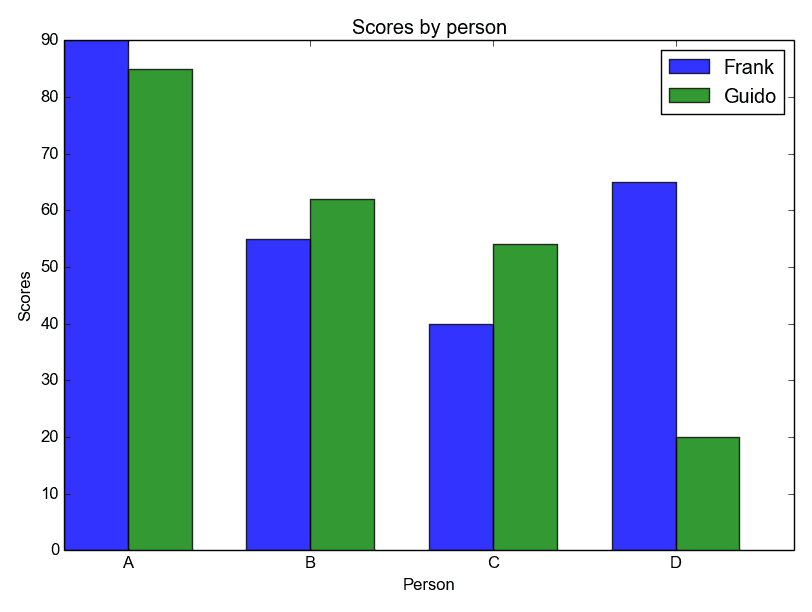# Tag: barchart

## Matplotlib Bar chart

Matplotlib may be used to create bar charts. You might like the Matplotlib gallery.

Matplotlib is a python library for visualizing data. You can use it to create bar charts in python. Installation of matplot is on pypi, so just use pip: pip install matplotlib

The course below is all about data visualization:

Related course:
Data Visualization with Matplotlib and Python

## Bar chart code

A bar chart shows values as vertical bars, where the position of each bar indicates the value it represents. matplot aims to make it as easy as possible to turn data into Bar Charts.

A bar chart in matplotlib made from python code. The code below creates a bar chart:

Output:Python Bar Chart

Matplotlib charts can be horizontal, to create a horizontal bar chart:

Output:Bar chart horizontal

## Bar chart comparison

You can compare two data series using this Matplotlib code:

Output:Python Bar Chart comparison

## Stacked bar chart

The example below creates a stacked bar chart with Matplotlib. Stacked bar plots show diffrent groups together.

Output: